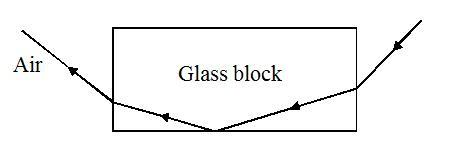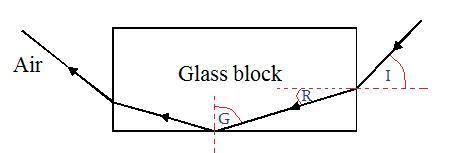# Incidence, Refraction, Critical Angle

## Homework Statement

Travellers in hot places often think that they see water in the distance, when there is nothing but land there. This effect is called a mirage. The air near the ground is very hot, and light reflects off the top of this layer of hot air. The diagram below shows how you could demonstrate the effect in a laboratory.On the diagram, draw appropriate normals and mark:
• An angle of incidence, labelled I,
• An angle of refraction, labelled R, and
• An angle labelled G which you know is greater than the critical angle.

2. The attempt at a solution^ Is it correct?

Hootenanny
Staff Emeritus
Gold Member

## Homework Statement

Travellers in hot places often think that they see water in the distance, when there is nothing but land there. This effect is called a mirage. The air near the ground is very hot, and light reflects off the top of this layer of hot air. The diagram below shows how you could demonstrate the effect in a laboratory.On the diagram, draw appropriate normals and mark:
• An angle of incidence, labelled I,
• An angle of refraction, labelled R, and
• An angle labelled G which you know is greater than the critical angle.

2. The attempt at a solution^ Is it correct?
Looks good to meI was confused if G was just as the ray left the glass block. So, it is correct, that it occurs during total internal reflection?

Hootenanny
Staff Emeritus
Gold Member
I was confused if G was just as the ray left the glass block. So, it is correct, that it occurs during total internal reflection?
I'm sorry I'm not sure what you mean. You know that G must be greater than the critical angle because TIR occurs.

So, is G the angle of incidence for total internal reflection to occur.

Isn't critical angle when incident angle equal reflected angle?Hootenanny
Staff Emeritus
Gold Member
So, is G the angle of incidence for total internal reflection to occur.

Isn't critical angle when incident angle equal reflected angle?No, all you can conclude from the diagram is that G is greater than the critical angle since we have TIR. Furthermore, the incident angle is always equal to the reflected angle.

If I drew G on the other side of the normal inside the glass block (where total internal reflection occurs), would that have been correct too? Or, is it always the angle from the normal line when it is incident ray?

Hootenanny
Staff Emeritus